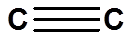# 1.9: sp Hybrid Orbitals and the Structure of Acetylene

Objectives

After completing this section, you should be able to

1. use the concept of sp hybridization to account for the formation of carbon-carbon triple bonds, and describe a carbon-carbon triple bond as consisting of one σ bond and two π bonds.
2. list the approximate bond lengths associated with typical carbon-carbon single bonds, double bonds and triple bonds. [You may need to review Sections 1.7 and 1.8.]
3. list the approximate bond angles associated with sp3-, sp2- and sp‑hybridized carbon atoms and predict the bond angles to be expected in given organic compounds. [If necessary, review Sections 1.6, 1.7 and 1.8.]
4. account for the differences in bond length, bond strength and bond angles found in compounds containing sp3-, sp2- and sp‑hybridized carbon atoms, such as ethane, ethylene and acetylene.

Key Terms

Make certain that you can define, and use in context, the key term below.

• sp hybrid orbital

Study Notes

The bond angles associated with sp3-, sp2- and sp‑hybridized carbon atoms are approximately 109.5°, 120° and 180°, respectively.

## Bonding in acetylene

Finally, the hybrid orbital concept applies well to triple-bonded groups, such as alkynes and nitriles. Consider, for example, the structure of ethyne (another common name is acetylene), the simplest alkyne.This molecule is linear: all four atoms lie in a straight line. The carbon-carbon triple bond is only 1.20Å long. In the hybrid orbital picture of acetylene, both carbons are sp-hybridized. In an sp-hybridized carbon, the 2s orbital combines with the 2px orbital to form two sp hybrid orbitals that are oriented at an angle of 180°with respect to each other (eg. along the x axis). The 2py and 2pz orbitals remain non-hybridized, and are oriented perpendicularly along the y and z axes, respectively.The C-C sigma bond is formed by the overlap of one sp orbital from each of the carbons, while the two C-H sigma bonds are formed by the overlap of the second sp orbital on each carbon with a 1s orbital on a hydrogen. Each carbon atom still has two half-filled 2py and 2pz orbitals, which are perpendicular both to each other and to the line formed by the sigma bonds. These two perpendicular pairs of p orbitals form two pi bonds between the carbons, resulting in a triple bond overall (one sigma bond plus two pi bonds).The hybrid orbital concept nicely explains another experimental observation: single bonds adjacent to double and triple bonds are progressively shorter and stronger than ‘normal’ single bonds, such as the one in a simple alkane. The carbon-carbon bond in ethane (structure A below) results from the overlap of two sp3 orbitals.In alkene B, however, the carbon-carbon single bond is the result of overlap between an sp2 orbital and an sp3 orbital, while in alkyne C the carbon-carbon single bond is the result of overlap between an sp orbital and an sp3 orbital. These are all single bonds, but the single bond in molecule C is shorter and stronger than the one in B, which is in turn shorter and stronger than the one in A.

The explanation here is relatively straightforward. An sp orbital is composed of one s orbital and one p orbital, and thus it has 50% s character and 50% p character. sp2 orbitals, by comparison, have 33% s character and 67% p character, while sp3 orbitals have 25% s character and 75% p character. Because of their spherical shape, 2s orbitals are smaller, and hold electrons closer and ‘tighter’ to the nucleus, compared to 2p orbitals. Consequently, bonds involving sp + sp3 overlap (as in alkyne C) are shorter and stronger than bonds involving sp2 + sp3 overlap (as in alkene B). Bonds involving sp3-sp3overlap (as in alkane A) are the longest and weakest of the group, because of the 75% ‘p’ character of the hybrids.

## Comparison of C-C bonds Ethane, Ethylene, and Acetylene

 Molecule Bond Bond Strength (kJ/mol) Bond Length (pm) Ethane, CH3CH3 (sp3) C-C (sp3) 376 154 Ethylene, H2C=CH2 (sp2) C=C (sp2) 728 134 Acetylene,(sp)(sp) 965 120

Notice that as the bond order increases the bond length decreases and the bond strength increases.

## Exercises

#### Questions

Q1.9.1

1-Cyclohexyne is a very strained molecule. By looking at the molecule explain why there is such a intermolecular strain using the knowledge of hybridization and bond angles.#### Solutions

S1.9.1

The alkyne is a sp hybridized orbital. By looking at a sp orbital, we can see that the bond angle is 180°, but in cyclohexane the regular angles would be 109.5°. Therefore the molecule would be strained to force the 180° to be a 109°.